## //----------------------------// ArtMatic 3 in 3 out components //----------------------------//

Introduction
3D Scale
3D Offset
3D Rotate
Normalise
3D Normal
3D Reflect
3D Spaces #
3D Repeats & Tile #
3D Plane Mirror
3D Mirrors & Rotates #
N-Fold Mirrors & Offset #
3D Distorts & Bend #
3D sphere
3D sphere B
3D plane
3D tube
3D parametric face
3D parametric plane
3D elevation slices
3D density slices
Conditional z set
3D Sines
3D Color techno noise
3D Color Mondrian
3D Color strata stone
3D Color Marble
3D Color Bubbles
3D Color spots
3D DFi patterns #
Saturation & Lum
Color Controls #
RGB Contrast
Color Shift
HLS to RGB
RGB to HLS
RGB gamma
RGB Gaussian
RGB dither
RGB Quantize
Memory Min
Memory Max
3D Random vector
3D Multi Perlin displace
3D Fractal displace
3D time turbulence
3D MultiFractal displace #
3D Perlin Vector Noise
3D Color Perlin noise
3D Color Fractal noise
3D Color MultiFractal
3D Caustics
Packed RGB Alpha
Packed RGB Max
Packed Maths #
Packed random Mix
Packed XYZ Depth sort
Packed XYA logic #
Memory Packed Alpha
Memory Diff
Memory Depth sort
33 Compiled tree

## Introduction

The components in this group cover a wide range of applications: 3D space distortion and object creation, color/light manipulation, and mixing. An essential in-depth discussion of function categories is found in the Getting Deeper chapter and is recommended reading for everyone. The inputs to the components are referred to as x, y, and z for the left, middle and right inputs. Vector packed values are reffered using upper cases X,Y, Z or A B C. Keep in mind that they may or may not actually be spatial coordinates. While many of these components were designed with particular applications in mind, it is worth exploring non-typical applications.

Tip: Try using the space manipulation components (rotate, sphere, etc.) as color manipulation tools. For example, insert Rotate or Sphere or 3D Random Vector components between RGB/HLS and HLS/RGB components to manipulate image color.
An essential in-depth discussion of ArtMatic structures Trees and components is found in ArtMatic Designer References and in Building trees.

##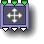33 3D Scale

parameters :
A : Scale x (-8. : 8.)
B : Scale y (-8. : 8.)
C : Scale z (-8. : 8.)

• Individual Axis (A,B,C)
With Individual Axis selected parameters A,B,C multiplies the inputs (x,y,z).
Formula: output vector v= ( A*x,B*y,C*z)
• Uniform Scaling
Uniform Scaling allows all dimensions to be scaled by the same amount set by parameter A. B and C can do addition scaling of y & z. Leave them at 1 for a strict uniform scaling.
Formula: output vector v= ( A*x,A*B*y,A*C*z)

##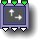33 3D Offset

parameters :
A : Offset x (-64. : 64.)
B : Offset y (-64. : 64.)
C : Offset z (-64. : 64.)

discussion :
Add an offset to the incoming values. Formula: xout = A + x yout = B + y zout = C + z

##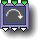33 3D Rotate

parameters :
A : Angle xy (oZ) (-180. : 180.)
B : Angle xz (oY) (-180. : 180.)
C : Angle yz (oX) (-180. : 180.)

discussion :
This component provides a few different methods of 3D rotations. While this component was designed to take a 3D space as its input, it can have an interesting affect when applied to color manipulation. Some algorithms provide both 3D rotation and 3D offset making them more efficient than the standard 3D Rotate plus an additional offset component. Component options allows to set the angle in radians or degrees. Existing values are remapped when changing the angle unit.

algorithms :
• Rotate 3 axes :
Parameters A, B and C provide the rotation amounts in the xy, xz and yz planes respectively.
• Rotate yz(oX) & Offset z & x :
Rotate the yz plane around the x axis. Parameter A controls rotation amount, and parameters B and C provide offsets along the z (depth) axis and x (horizontal) axis (the axis of rotation) respectively.
• Rotate xz(oY) & Offset x & y :
Rotate the xz plane around the y axis. Parameter A controls rotation amount, and parameters B and C provide offsets along the x (horizontal) axis and y (vertical) axis.
• Rotate xy(oZ) & Offset y & z :
Rotate the xy plane around the z axis. Parameter A controls rotation amount, and parameters B and C provide offsets along the y (vertical) axis and z (depth) axis.

##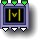33 Normalise

parameters :
A : Magnitude (0.50 : 8.)

discussion :
This is a mathematical primitive that normalizes the incoming 3D vector(x,y,z) by dividing it with its length. The vector's magnitude is set to unity and maintains its orientation. The 'Magnitude' parameter scales the resulting vector magnitude.

Formula: Returns the vector (xM,yM,zM) with M = A/sqrt(x^2 + y^2 + z^2)

##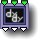33 3D Normal

parameters :
A : Magnitude (0. : 4.)

discussion :
3D Normal uses finite differences to calculate the orientation of the incoming space/object. The normal is the vector perpendicular to the surface's tangent plane. 3D Normal generally needs to be followed by a dot-product with light vector to yield a meaningful lighting effect (see
Illumination). Using this component directly as an RGB-shader creates the illusion that red, green and blue lights are illuminating the object.
Parameter A controls the Magnitude of the Normal vector and should be left at 1 in most cases.
The derivatives are taken by evaluating the tree above several times. Thus this component has to "see" the function above which means it cannot be used within a CT to compute the derivative of a function depending on values computed outside. Practically if you create a CT for a function and needs its derivative, put the derivative component outside the CT and connect the derivative to the CT output.
When used with loopers the derivative component shall be placed outside the loop, that is, after a memory component like 33 Memory Max.
Note: This component has been reimplemented in ArtMatic engine 8.07 to provide a more exact normal, in particular with pseudo 3D primitives. It is meant to be used with 3D vector valued function only. To get the 3D Normal of a 3D scalar field or a 2D surface, use the 13 3D derivative (dx,dy,dz) component.

##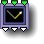33 3D Reflect

parameters :
A : Viewvec scale (0. : 2.)

discussion :
Implemented in ArtMatic engine 8.07, 3D reflects simplifies the implementation of reflection shaders. It takes a 3D vector as input (usually a 3D Normal) and returns a reflected 3D vector relative to an implicit view vector V. The reflected vector is computed as R= V - 2*V.N * N, N being the incoming Normale vector. The 'Viewvec scale' parameter determines how narrow is the virtual viewport which is oriented in z and will use global x & y canvas coordinates independently of canvas zoom and position. The reflected output vector can be fed to a 360° environment picture with a spherical projection or a procedural 3D function to define the reflection shading content. ArtMatic pseudo 3D objects like
3D plane can reflects things correctly when using the 3D Normal component described above.

Example files : Libraries/Components 8.07/2D surface DEnvRef, Libraries/Components 8.07/3D objects DEnvRef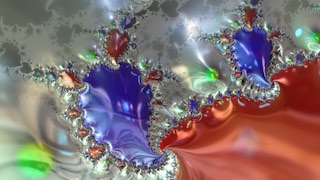Reflective Julia fractal. (Libraries/Components 8.07/F OAN DEnvRef Shaded.artm)

##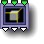33 3D Spaces #

parameters :
A : Rotation (d) (-180. : 180.)
B : Translate X (-32. : 32.)
C : Translate Y (-32. : 32.)
D : Translate Z (-32. : 32.)

discussion :
This component provides some useful preset 3D spaces that are especially useful for creating DFRM objects. Parameters may change depending on the Algorithm.
Parameter Options note: orient +, orient -, orient + and offsets*10, orient - & offsets*10. The "*10" options are useful when you need offsets larger than the provided range. The +/- options only have significance when the algorithm uses absolute value, such as Bilateral Split |y|.

See the series of examples provided with ArtMatic Voyager to get some idea of how it is used.
ArtMatic Voyager CTX/Voyager Examples/DF Modelling/
ArtMatic Voyager CTX/Voyager Examples/Components/DF 44 Spaces/

algorithms :
• Translate & Rotate oY:
• Translate & Rotate oZ:
• Translate & Rotate oX:
Rotates the space on the given axis and translate by the translate (x,y,z) parameters.
• Normal space xy, rotation oY:
Normal orientation is preserved but the space is rotated around y axis and offset according to the parameters. Unlike Translate & Rotate oY the offsets are only provided for depth and main axis and no changes is at 0.5.
• Top view space xz, rotation oZ:
This flips the space 90 degrees vertically so that you have a top view of the object. The effect is the same as using 33 Rotate to rotate yz by 90° (π/2).
• Side view space zy, rotation oX:
This flips the space 90 degrees on its side so that you have a side view of the object. The effect is the same as using 33 Rotate to rotate xy by 90° (π/2).
• Artmatic top view space :
This mode allows you to quickly switch to a top-view of the space without having an impact on ArtMatic Voyager (which will use the normal view). This algorithm is quite useful if you are working on details and a top-view would be useful in ArtMatic but where you do not want to change the object's orientation in ArtMatic voyager.
• Mirror x & Translate:
Mirror the space on the x axis and translate by the translate (x,y,z) parameters.
• Mirror y, Rotate oX & offset z:
• Mirror y, Rotate oZ & offset x:
• Mirror x, Rotate oY & offset z:
• Mirror x, Rotate oZ & offset x:
Mirror the space on the x or y axis and provides various combination of rotation axis and translations. Parameters 'Mirror offset' shall usually remain at zero while 'offset' translate in the same axis than the mirroring. 'Smoothness' parameter smooth the mirror edge.
• Mirror All axis:
Mirrors the space in all directions by taking the absolute value of all axes. 'Smoothness' parameter smooth the mirror edge.
• Bilateral split |y|:
• Bilateral split |z|:
Theses create a symmetrical bifurcated space mirrored along the axis in the name. This component is useful for creating rotated mirror copies or to spit an element into two branches. The two spaces are offset by amount C and then rotated. When A is 0.5, the result is just a symmetrical space. The resulting space is not continuous which can be problematic for DFRM if the shapes are not placed correctly, that is away enough from the region where the space splits.
• Orientable |y| & rotate oZ :
• Orientable |y| & rotate oX :
Theses two algos mirrors the space in y, rotates it in the given axis and re-orients everything by the amount specified by parameter 'Orientation'.
• Mirror & offset x, floor & Rotate oZ :
• Mirror & offset y, floor & Rotate oX :
Mirror the space on the x or y axis with negative parts being clamped to zero and provides various combination of rotation axis and translations. Practically the floor operation creates a connection between either side of the mirror when the offset spread them apart.

##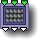33 3D Repeats & Tile #

parameters :
A : Tile X % (0. : 1.)
B : Tile Y % (0. : 1.)
C : Tile Z % (0. : 1.)

discussion :
Formerly 3D Tile, this component provides several flavors of 3D Tiling and repetition. It is useful for creating regular tilings of space which can be useful for creating 3D objects that feature repeating patterns. The Cell Radius parameter defines the spacing between the repetitions. The repetition parameters set the number of repeats along the specified axis. For some algorithm, a fourth parameter is available and specifies an optional randomized rotation for the repeats. This rotation is useful for creating sets of plants or other objects that you don't want to look identical.

Notes: The produced space is not continuous at cell boundaries. So, care should be taken that objects don't extend to or beyond the cell boundary. You may need to use the value*10 parameter option to set the cell radius.

Parameters: A - Tile X, B - Tile Y, C - Tile Z. The parameter range for A, B and C is 0 to 1. The parameter value sets the spacing of the tiling along the associated axis. When the parameter is 0 , there is no tiling along that axis.

Parameter options:

• Use 2/period. This option treats the parameter value as frequency. Low values increase distance while high values decrease it. At 1, the tiles overlap slightly.
• Use period. Treats the parameter as the distance between the tiles.
Example: Overhead view of the line, rect, cross and ring sets using a disk for each instances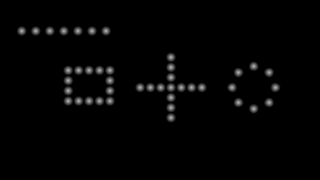Example: Overhead view of Array, Arch & Corners sets using a disk for each instances.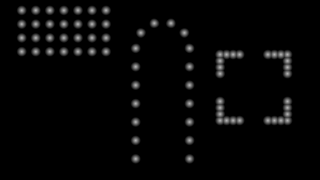Example: This Arcade element repeated with the Ring algorithm.Example: This Arcade patio uses the Corner mode to repeat the arcades.Algorithm Notes: The Infinite and Repeats algorithms repeat the input cell in a three dimensional pattern with parameters A-C setting the spacing along the three axes. The Sets algorithms are useful for architectural design and modeling. They deposit copies of the input cell along the path shape described by the algorithm.

algorithms :
• Infinite Tile :
Repeat infinitely. Parameters A-C set the distance between repetitions along x, y and z respectively. By default, repeats are along the x axis. When the parameters equal zero, no repeats are performed in that dimension.
• Repeats N (mod):
Repeat a finite number of times with the number of times determined by parameter D. Parameters A - C determine the spacing for the x, y and z axes just as in Infinite Tile mode.
• Sets xz : line :
• Sets xz : Rect :
• Sets xz : Cross :
• Sets xz : Array :
• Sets xz : Ring :
• Sets xz : Arch :
• Sets xz : Corners :
• Fold N (saw) :
• Cluster Ring xz :
• Cluster Ring xy :
• Cluster Ring+Center xz :
Each space cell is placed on N division of a circle in the xz plane.
• Cluster Ring+Center xy :
Same as Cluster Ring but with the object placed also in the disk center. You will get N+1 duplicates. For all Cluster Rings Parameters C sets a delta of displacement on a complement axis & Parameters D randomizes the offset in the 2 axis of the ring.
• Cluster Line x :
Each space cell is placed on N division of a Line along the x axis. Y & z positions can be randomized with parameters C & D.
• Cluster Line z :
Each space cell is placed on N division of a Line along the z axis. X & y positions can be randomized with parameters C & D.

##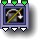33 3D Plane Mirror

parameters :
A : Plane Nx (-2. : 2.)
B : Plane Ny (-2. : 2.)
C : Plane Nz (-2. : 2.)
D : Offset (-50. : 50.)

discussion :
3D Plane Mirror implements a mirroring of space in respect with a plane that can be oriented by its normal. The Normal is set by Parameters Nx,Ny,Nz. A ground plane will be (0,-1,0) while a plane oriented in x will have (1,0,0) as its normal. The plane can be translated in space by parameter 'Offset'. Plane Mirror is a very useful tool for DF modeling and its the 3D equivalent of the
2D Mirror tile.
Mirroring can be used for creating directional symmetries, 3D fractals, and a wide range of shapes and architectures as demonstrated by the examples in the DF Modeling Voyager folder.

algorithms :
• hard mirror:
No smoothing at the mirror edge
• smooth mirror:
Mirror edge slightly smoothed
• curved mirror,
• wider curved mirror :
Mirror edge replaced by a curve of medium and wide radius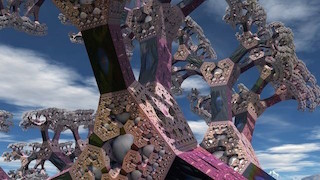3D fractal example based on 2 Plane mirrors and 2 abs.

##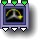33 3D Mirrors & Rotates #

parameters :
Algorithm slider : (0 : 19)
B : N rotate (2 : 32)
C : Angle (0. : 90.)

discussion :
This component provides several 3D space transformations that are especially useful for 3D objects modeling. The transforms include a variety of rotation and mirroring algorithms along with tools specific for plants like randomized spread or bendings.

Many primitive plants and animals have a rotational symmetries and Mirrors and Rotates is a practical tool to implement theses types of structures. For more complex plant structures you may also use the
34 Jitter Axial and Jitter Spherical components or even combine them with 3D Mirrors and Rotates.

3D Mirrors and Rotates is a pretty useful for architecture and ornamental design. See also the 2D version of rotational symmetries N-Fold Mirrors and Branch N.

Example: Alien Plant using Rotate N xz & Spread & Bend Up with N=9 rotations and an angle of 48 degree. The randomized spread makes branch of different heights and the bending gives the plant a more natural look.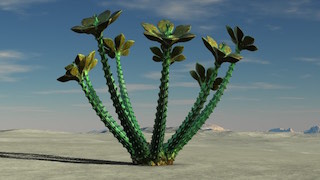Example: Same Plant using Rotate N xz & Branch 3 with N=4 rotations and an angle of 23 degree. The Branch 3 subdivides the space to yield 3 sub branches.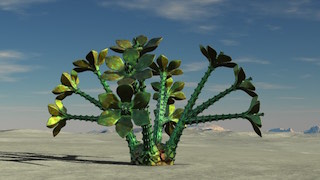Example: Same Plant usingBilateral(x) Rotate N xy with N=5 rotations and an angle of 86 degree (other branches are rotated downward).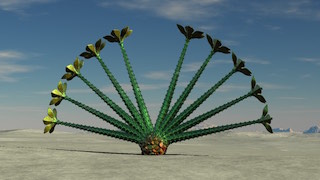algorithms :
• Mirror N xy sharp :
• Mirror N xy smooth :
Mirror each tile/branch and perform N rotations using rotational symmetries with respect to the xy plane.
• Rotate N xy:
N rotations with in the xy plane (standing). In this case the rotational symmetries
• are without mirroring on the central axis causing the space to be highly discontinuous on the branches edges.
• Mirror N xz sharp:
• Mirror N xz smooth:
Mirror each tile/branch and perform N rotations using rotational symmetry with respect to the xz plane.
• Rotate N xz:
N rotations with in the xz plane (ground).
• Helix in Y:
Create a helical transformation of the space with respect to the y axis.
• Mirror N xz & Rotate 30 zy:
Rotational symmetry with respect to the xz plane plus a rotation of 30° in the zy plane.
• Mirror N xz & Rotate -30 zy:
Rotational symmetry with respect to the xz plane plus a rotation of -30° in the zy plane.
• Mirror N xz & tilt C y:
• Mirror N xz & tilt -C z:
Mirror symmetry of the input with radial symmetries with respect to the xz axis plus a rotation of -C° in the zy plane. Parameter C "Angle" controls the vertical rotation in this algorithm (while in algorithms 0 through 8 it controlled the offset). The upper example shows C = 2 while the lower example has C=60.
• Rotate N xz & Spread:
Radial rotations without mirroring plus a randomized offset for each rotation. Parameter C controls the angle by which the rotations are spread.
• Rotate N xz & Spread & Bend Up:
• Rotate N xz & Spread & Bend Down:
Radial rotations plus a randomized Spread offset for each rotation with a bending up or down. Great for small DF plant design.
• Rotate N xz & Branch 3:
With N=4 rotations and an angle of 23 degree. The Branch 3 subdivides the space to yield 3 sub branches.
• Rotate N xz Random:
xz symmetries with randomized rotations and offset with a spread of -C° in the zy plane.
• Bilateral(x) Rotate N xy:
• Bilateral(x) Rotate N xy & spread:
• Bilateral(x) Rotate N xz:
• Bilateral(x) Rotate N xz & spread :
This series uses a bilateral symmetry prior the rotations folding in the xy or xz plane. They are good to create fan like structures plants. No bending is included there so you may want to put an additional displacement tile like the 3D Distorts and Bends explained below to bend the space prior using 3D Mirrors and Rotates #

##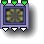33 N-Fold Mirrors & Offset #

parameters :
A : Rotation (d) (-180. : 180.)
B : Offset x (-100. : 100.)
C : Offset y (-100. : 100.)
D : Offset z (-100. : 100.)

discussion :
This component implements
rotational symmetries with mirroring. It is similar to Mirrors & Rotates but offers a choice of orientations and higher ranges for displacements.
Parameters: 'Offsets' (x,y,z) provide post-rotation displacements in each dimension. Parameter 'Rotation' sets the pre-folding rotation of the space. The number of symmetries N is defined by the Options pop up.
The resulting space has N symmetrical branches. This component is often used with 21 Profile Shapes # or 34 Uvid Sweep Volumes.

algorithms :
• xy plane NFold mirror x+ :
• xz plane NFold mirror x+ :
• yz plane NFold mirror y+:
• xy plane NFold mirror x-:
• yz plane NFold mirror y-:
• xz plane NFold mirror z-
• The various algorithms select the rotation plane and the mirroring orientation. Use 'xz' for vertical structures folding.

##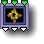33 3D Distorts & Bend #

parameters :
Algorithm slider : (0 : 32)
B : Amount (-2. : 2.)
C : Scale / period (0. : 16.)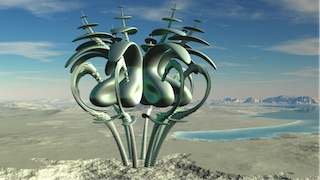Note: Algorithm Name Conventions use the following conventions in their names. A capitalized X, Y or Z indicates an axis of motion or orientation. A lower-case x,y or z indicates a direction that only applies if the appropriate parameter option is selected. For example, Undulate Y in Zx indicates that there will be undulations of the Y direction (vertical) along the Z (depth) axis and that there can be optional motion along x (the horizontal direction).

algorithms :
• Dist xz ripples y :
Distort the space vertically using formula: sin(dist* scale) * amount.
• Dist xz bend y:
Bend the space vertically by an amount that is a function of the distance to to the center (0) of the xz plane.
• Xy plane bend :
Bend the space in the direction opposite to the given plane. As bend is in essence non linear it may distort the space too much for proper DFRM convergence. Use it with caution using low bending amounts.
• Zy plane bend :
• Yx plane bend :
Bend the space in the direction opposite to the given plane. As bend is in essence non linear it may distort the space too much for proper DFRM convergence. Use it with caution using low bending amounts.
• Rotate xz by y :
Vertical twirl of the space by amount * scale.
• Warp y :
Distort the space's scale on the y axis.
• Warp Dist :
Distort the space proportional to its distance from the center (0).
• Cylindrical bumps (oY) :
• Cylindrical & y bumps (oY) :
• Cylindrical & y spikes (oY) :
• Cylindrical randomize (oY) :
Cylindrical coordinate displacement pattern (angle(x,z),y). This works nicely for objects of revolution and branches. Strong displacements can result in poor convergence. If there are artifacts even at Best and Sublime quality, the amount of distortion should be reduced.
• Spherical bumps :
• Spherical spikes :
• Spherical ripples :
Spherical coordinate displacement patterns. Equations: (angle(x,z),angle(y,dist))
• Undulate Y in Zx :
There are now algorithms to undulate shapes over time which is essential for animating creatures without having to keyframe every motion. Vertical undulations that proceed along the z axis (depth). In other words, undulations up and down that move from front to back when applied to and object aligned with the z (front/back) axis. With the sideways option, it can be used to undulate lateral fish fins.
• Undulate Y in Zy :
Vertical undulations with 'sideways' option applying to y it can be used to undulate dorsal fish fins.
• Undulate X in Zy :
Snake-like left-right undulations proceeding from front to back.
• Undulate X in Yz :
Back-and-forth (left/right) undulations of the vertical axis such as you might use for undulating vertical tentacles or seaweed.
• Undulate Z in Yx :
Front/back undulations along the vertical axis, such as you might use for undulating vertical tentacles or seaweed.
• Undulate radially in Yx :
• Undulate radially in Zx :
Radial undulations create displacements radiate from the center and move along the named axis.
• Undulate spherically Yx :
Undulates radiate outwards spherically from the origin.
• Random Displace X :
• Random Displace Y :
• Random Displace Z :
Random displacement along the given axis using low frequency noise animated over time.
• Polar sines X :
• Polar sines Y :
• Polar sines Z :
The 3 polar sines algorithms undulate radially perpendicular to the main axis. For example, Polar Sines Y undulate radially in the xz plane. These undulations are not automatically animated.
• Time ripples xy in Z :
Automatically animated ripples in the xy plane that radiate from the Z axis.
• Time ripples xz in Y :
Automatically animated ripples in the xz plane that radiate from the Y axis.
• Power bend z in |Y| :
Bends an object in the Z direction (depth) with the amount of bending determined by 2y. This algorithm can be useful for bending a triangular dorsal fin into a more natural shape, for example. The amount should be set carefully to avoid problems with convergence.
• Power bend y in |X| :
Bends an object in the Y dimension (height) with the amount of bending being determined by 2x. This algorithm can be useful for bending lateral fins up and down on a z-oriented fish, for example.

##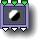33 3D sphere

parameters :
A : Angle xz (-12.57 : 12.57)
B : Angle yz (0. : 2.)
C : Displace z (0. : 1.)

discussion :
Renders a pseudo 3D sphere that stick out of a plane located at z origin. It returns the 3D coordinates of the sphere and the plane surface. This 3D object behaves a bit differently than the other 3D objects : infinity is not used as the value for the regions outside of the sphere. The sphere appears against the same background that provides its surface texture. The object is rendered on the (x,y) canvas and the extra z input is used only to distort the space.

##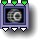33 3D sphere B

parameters :
A : Offset x (-16. : 16.)
B : Offset y (-16. : 16.)
C : Offset z (-16. : 16.)

##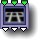33 3D plane

parameters :
A : Banking X (-1.57 : 1.57)
B : Plane Offset (-16. : 16.)
C : Z slope (-3.14 : 3.14)

discussion :
Create a 3D plane which can be banked and tilted. Like all 3D object components, the area outside the object is mapped to infinity (which is painted with the first auxiliary color -- the
Depth cue color.) The components which follow the plane component provide the surface's texture. Banking x controls the horizontal tilt, Plane offset provides an altitude offset, and z Slope controls the forward/back tilt.

##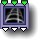33 3D tube

parameters :
A : Offset x (-16. : 16.)
B : Offset y (-16. : 16.)
C : Radius (1. : 12.57)

discussion :
Similar to the 23
3D tube this version has an additional input z that modulates the distance to the virtual "camera".

##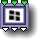33 3D parametric face

parameters :
A : Angle xy (-Π2 : Π2)
B : Angle zx (-Π2 : Π2)
C : Offset z (-32. : 32.)
D : Height (-3 : 3)

discussion :
Similar to the 23
3D parametric face this version has an additional input z that modulates the distance to the virtual "camera". A non-constant z input will cause the face to be distorted. ArtMatic Engine 8.0 provides a new parameter 'Height' to set the aspect ratio of the face. Use zero 'Height' to get a square.
See 3D plane for more details on pseudo 3D objects.

##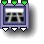33 3D parametric plane

parameters :
A : Angle xy (-Π2 : Π2)
B : Angle zx (-Π2 : Π2)
C : Offset z (-32. : 32.)

discussion :
Similar to the 23
3D plane this version has an additional input that modulates the distance to the virtual "camera". The z value can set by the Iteration component when you need a series of 3D parallel planes. A non-constant z input will cause the plane to be distorted. The x & y outputs should be used for 2D texturing. Unlike 3D parametric face above the plane is infinite in dimension.

##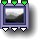33 3D elevation slices

parameters :
A : Scale (0.25 : 8.)
B : Slope (0. : 4.)
C : Offset z (-32. : 32.)

discussion :
This is an advanced component that makes certain kinds of 3D modeling possible. This component can be used to visualize 2D surfaces or terrains by iteratively drawing slices over the z-axis. Since true 3D modeling is beyond ArtMatic's current capabilities, this component was added to simulate 3D rendering of some systems. The third input must be connected to a 21 function to be evaluated or to a 21 compiled tree. The first two inputs determine the points to be evaluated. Scale acts as a magnification control. Slope controls angle of view of the 3D space. Offset controls the spacing of the slices on the z axis.

Examples: Look for the word "elevation" in the examples library for examples of this component.

##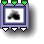33 3D density slices

parameters :
A : Scale (0.25 : 4.)
B : Surface level (0.12 : 4.)
C : Offset z (-16. : 16.)

discussion :
Another component for simulating 3D modeling. This component works by taking an input function and sampling it iteratively over the z-axis. It is a primitive sort of volumetric rendering. The slices are limited to a plane bounded by -Pi and +Pi on the z-axis. The third input must be connected to a 31 component which will be the function to evaluated iteratively.

Examples: Look for the word "density' in the examples library for examples of this component.Memory Functions The next several components (all with Memory in their names) are intended for use in (and are only meaningful in) iterative systems which are discussed in detail in the chapter Compiled Trees and Iteration.

##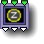33 Conditional z set

parameters :
A : Threshold (0. : 32.)
B : Minimum (0. : 1.)

discussion :
This is an advanced component that can be used to create "levelset'-type shading when implementing classical-style fractals such as the Mandelbrot or Julia sets. When the distance (of the x, y inputs from the origin (0, 0)) is above the Threshold parameter or below the Minimum parameter the incoming z value is passed to the third outlet otherwise 0 is sent out the third outlet. x and y are passed through unchanged to the left and middle outlets. Hence, when x,y is within a specific range, the third outlet is non-zero and can be used for shading.

Generally, the z-input will be connected to the output of the Iteration component in a system with memory components. In such a system, it is possible to color a point whose value is infinity with a color related to the number of iterations required to reach infinity. This component can also be used to create distance masking as in the Clock example file.

##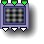33 3D Sines

parameters :
A : Amplitude (0. : 8.)
B : Phase (-32. : 32.)
C : Frequency (0.03 : 16.)

discussion :
3D math primitive which generates sin(x) in the red channel, sin(y) in the green channel and sin(z) in the blue channel. This periodic component is equivalent to three parallel 1D sine filters.

##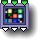33 3D Color techno noise

parameters :
A : Amplitude (0. : 8.)
B : Phase (-32. : 32.)
C : Frequency (0. : 16.)

discussion :
An RGB version of the 31 3D Techno Noise.

##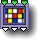33 3D Color Mondrian

parameters :
A : Line Width (0. : 1.)
B : Phase (-32. : 32.)
C : Frequency (0. : 16.)

discussion :
Computes a color 3D texture inspired by Mondrian paintings with primary colors in rectangular random arrangements.

##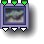33 3D Color strata stone

parameters :
A : Chroma (0. : 1.)
B : Distortion (-2. : 2.)
C : Frequency (0. : 8.)

discussion :
RGB version of the 31
3D Stratified noise. The 'Chroma' parameter interpolates between grayscale and colored output. Parameter 'Distortion' controls the amount of vertical distortion.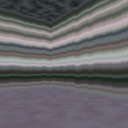##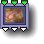33 3D Color Marble

parameters :
A : Hue Rotation (0. : 1.)
B : Saturation (0. : 1.)
C : Frequency (0. : 8.)

discussion :
3D color shading function that creates a veined, simple marble-like texture. 'Hue Rotation' controls the tint of the resulting texture while 'Saturation' controls the color saturation, and 'Frequency' controls the spacing of the veins.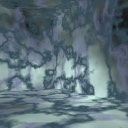##33 3D Color Bubbles

parameters :
A : Amplitude (0. : 1.)
B : Phase (-32. : 32.)
C : Frequency (0. : 8.)

discussion :
RGB shading function that provides randomly shaped and colored bubbles.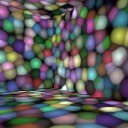##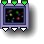33 3D Color spots

parameters :
A : Chroma (0. : 1.)
B : Phase (-32. : 32.)
C : Frequency (0. : 16.)

discussion :
An RGB version of the
31 3D Spots noise function. The 'Chroma' parameter interpolates between grayscale and color output.

##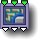33 3D DFi patterns #

parameters :
A : Thickness (-1. : 1.)
B : Phase (-32. : 32.)
C : Frequency (0.03 : 32.)
D : Smoothness % (0. : 1.)

discussion :
This new tile produces 2 DF fields and one index value. It was added to provide a texturing solution for 2 DF output tiles like the DF cities, DF buildings, DF polyhedron and constructs. The index can be used to switch between various colors or textures. The idea for 2 DF output is to allow separate shading for different elements, typically here window and structures.

The texture provides details information that typically will be added to a volume using logic tools # "bump intersect" or "partial subtract".

Example: Building Pattern B applied to a 3D DF Surfaces.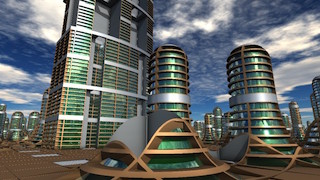Example:"Star Ship pattern P" for Sci Fi constructions and ships.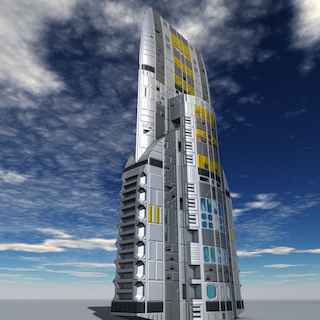algorithms :
• building pattern A :
• building pattern B :
• building pattern C :
• building pattern D :
• building pattern E :
• building pattern F :
• Cross45 building pattern G :
• building pattern H :
• building pattern I :
• Arch building pattern J :
This algorithm has changed. It now uses spherical arches so that they stay arches in whatever cutting direction
• building pattern K :
Fixed some continuity issues and improved the field quality.
• Ship pattern L :
A texture designed for Sci Fi constructions and ships.
• Space station pattern N :
• Random spheres pattern M :
• Windows & maze pattern O :
• Star Ship pattern P :

##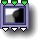33 Max to gradient

parameters :
A : Amplitude X (0. : 2.)
B : Amplitude Y (0. : 2.)
C : Amplitude Z (0. : 2.)

discussion :
Treat the three inputs as three surfaces (fx, fy, fz). For each point, the output is the maximum of fx, fy and fz. If the maximum is fx, the left part of the gradient provides the color. If the maximum is fy, the middle of the gradient is used. If the maximum is fz, the righthand part of the gradient is used. The Amplitude parameter sets the range of colors of the gradient that is used (with a value of 0 a constant color is used for each area). For more information and an illustration, see the discussion of the 23 version of this component.

Note: Max to Gradient was designed to be used with ArtMatic Voyager to provide colors for a surface based on the maximum of the inputs (as with the 3/1 MinMax) component. Each "winner" of the maximum will have its own color.

##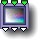33 Saturation & Lum

parameters :
A : Saturation (0. : 1.)
B : Brightness (0. : 2.)

discussion :
This component provides direct control of the saturation and luminosity of the incoming RGB values. The output values are restricted to a valid range. (No negative values are passed out).

##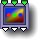33 Color Controls #

parameters :
Algorithm dependent

discussion :
This component provides various useful algorithms for color adjustment and color filtering:

algorithms :

##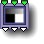33 RGB Contrast

parameters :
A : Contrast amount (-1. : 1.)
B : Offset (-4. : 4.)

discussion :
A component for controlling the contrast of RGB images. When the Contrast parameter is 0, there is no effect. At the maximum value, there are only two output values. The offset parameter controls the contrast divide point. With RGB output, the offset adjusts the overall luminosity. Colors are clamped to valid ranges (no negative values).

##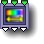33 Color Shift

parameters :
A : Hue shift (-6. : 6.)
B : Lightness % (-2. : 2.)
C : Saturation % (0. : 2.)

discussion :
Like the 44 version, this component controls hue, saturation and luminosity and clamps the colors to valid nominal ranges (0 - 8).

##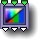33 HLS to RGB

parameters :
A : Contrast Hue (0. : 2.)
B : Contrast Luminance (0. : 2.)
C : Contrast Saturation (0. : 2.)

discussion :
Colorspace function that converts an HLS-based color stream back to RGB. This component is almost always used at some point following an RGB to HLS component. By inserting components between this component and the HLS to RGB component, you can manipulate the hue, luminance and saturation of an image which make many interesting color manipulations possible.

##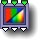33 RGB to HLS

parameters :
A : Amplitude (0.50 : 2.)

discussion :
Colorspace function that converts RGB-based color to HLS (hue, luminance, saturation). By inserting components between this component and the HLS to RGB component, you can manipulate the hue, luminance and saturation of an image which make many interesting color manipulations possible.

##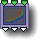33 RGB gamma

parameters :
A : Gamma red (0.12 : 8.)
B : Gamma green (0.12 : 8.)
C : Gamma blue (0.12 : 8.)

discussion :
Color manipulation function that provides independent control of the gamma (color response curve) of the red, green and blue channels. No changes are taking place when Gamma red green blue parameters are (1,1,1). Alternatively you can use RGB gamma to apply three power function in parallel.

##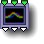33 RGB Gaussian

parameters :
A : Offset x (-16. : 16.)
B : Offset y (-16. : 16.)
C : Offset z (-16. : 16.)
D : Scale (0. : 32.)

discussion :
A gaussian filter is applied in parallel to the (x,y,z) inputs. The Offset parameter for each input displaces the center position of the gaussian curves while 'Scale' parameter (engine 8.07) sets the width of the bell curve. RGB gaussian creates symmetrical red, green and blue gaussian bands.

##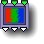33 RGB dither

parameters :
A : Amplitude (0. : 10.)

discussion :
Add high frequency random noise to the RGB inputs. When using direct RGB systems (systems with 3 outputs), RGB dither can be added at the end of the tree before the final quantization into 8 bit per channel RGB to minimize banding and color quantization artifacts. This function's noise algorithm is independent of the canvas' current zoom and position.

##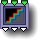33 RGB Quantize

parameters :
A : Frequency (0.12 : 4.)
B : Phase (-4. : 4.)
C : Amplitude (0. : 1.)

discussion :
Similar to the
11 Quantize component but operates on all inputs simultaneously. When applied to RGB data, it reduces the number of output colors and creates a posterisation effect.

##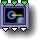33 Memory Min

parameters :
No parameters.

discussion :
This memory component stores the minimum of the red, green and blue of the current iteration and the stored values. It is used in looped/iterated/recursive trees to combine the results of each pass through the loop. Infinities are transmitted and incoming infinities are treated as transparent.

recursion formula:
mem_red =Min(red,mem_red)
mem_green=Min(green,mem_green)
mem_blue =Min(blue,mem_blue)

##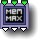33 Memory Max

parameters :
No parameters.

discussion :
This memory component stores the maximum of the red, green and blue of the current iteration and the stored values. It is used in looped/iterated/recursive trees to combine the results of each pass through the loop. Infinities are transmitted and incoming infinities are treated as transparent.

recursion formula:
mem_red =Max(red,mem_red)
mem_green=Max(green,mem_green)
mem_blue =Max(blue,mem_blue)

##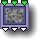33 3D Random vector

parameters :
A : Amplitude (-4. : 4.)
B : Phase (-32. : 32.)
C : Frequency (0. : 32.)

discussion :
3D space distortion function. Place this after a 3D object to provide surface texture. If you place a noise component before a 3D object, you will warp the space in which the object exists (and thus warp the object). This is a distortion-type noise whose output is made up of randomized modulations of the input values.

##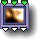33 3D Multi Perlin displace

parameters :
A : Amplitude (-8. : 8.)
B : Octaves (1. : 128.)
C : Frequency (0. : 32.)
D : Roughness (0.25 : 0.75)

discussion :
This component sums a series of 3D Perlin noise functions with the frequency doubling for each function in the series. The maximum value for the 'Octaves' parameter is 128 (2^7) and provides seven "octaves". This component allows very fine control of the frequency content. It is especially useful when used with very low frequency values to create surfaces for ArtMatic Voyager. See also 2D Multi Perlin noise and the 31 scalar version of 3D Multi Perlin noise.
'Frequency' abides to the standard
frequency options while 'Amplitude' controls the displacement amount. 'Roughness' adjust the fractal dimension : at 0.5 amplitude is divided by 2 when frequency is multiplied by 2. 'Roughness' has an effect only when more than 2 octaves are present.

##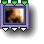33 3D Fractal displace

parameters :
A : Amplitude (0. : 8.)
B : Phase (-32. : 32.)
C : Frequency (0. : 32.)
D : Roughness (0.25 : 0.75)

##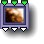33 3D time turbulence

parameters :
A : Amplitude (0. : 8.)
B : Feedback (-12. : 12.)
C : Frequency (0. : 32.)
D : Pace (0. : 10)

discussion :
A 3D fractal vector displacement function with feedback. The feedback parameter causes displacements of lower frequencies to be added to the higher frequencies. It is a good primitive for 3D fractal flow and smoke. This component is automatically modulated by time (even when locked). Parameter D, and Pace control the speed of the time-related animation.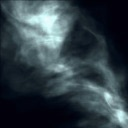##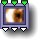33 3D MultiFractal displace #

parameters :
A : Amplitude (0. : 8.)
B : Mutation % (0. : 1.)
C : Frequency (0. : 32.)
D : Roughness (0.25 : 0.75)

discussion :
This 3D MultiFractal vector displacement function provides several algorithms listed below.
'Frequency' abides to the standard
frequency options while 'Amplitude' controls the displacement amount. 'Roughness' adjust the fractal dimension : at 0.5 amplitude is divided by 2 when frequency is multiplied by 2.

algorithms :
• Fractal Power displace :
The 3D displacement equivalent of 21 Fractal noises # (Fractal Power).
• Fractal Cliffs displace :
Similar to 21 Fractal noises # (Fractal cliffs) , Fractal Cliffs displace uses a smooth clamp function on the basic perlin noise to create sharp slope and flat areas.
• Multifractal displace :
The 3D displacement equivalent of 21 MultiFractal noise

##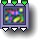33 3D Perlin Vector Noise

parameters :
A : Amplitude (0. : 8.)
B : Phase (-32. : 32.)
C : Frequency (0. : 32.)

discussion :
Returns the absolute value of three independent 3D vector Perlin Noise functions. It is a useful primitive for creating random RGB gradient curves. Use the phase parameter to select the colors.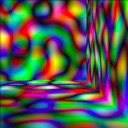##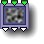33 3D Color Perlin noise

parameters :
A : Hue Rotation (-1. : 1.)
B : Saturation (0. : 1.)
C : Frequency (0. : 8.)
D : Variance (0. : 8.)

discussion :
A color texture based on scalar 3D Perlin noise. 'Hue Rotation' changes the main hue, and 'Saturation' sets the saturation of the colors. A zero value will give you a gray scale noise.
'Variance', introduced in ArtMatic engine 8.06 controls how many hues will be used to color the noise.
Tip : Connecting the z input to global time will animate the texture independently of keyframes presence.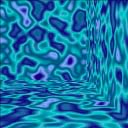##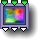33 3D Color Fractal noise

parameters :
A : Amplitude (0. : 8.)
B : Phase (-32. : 32.)
C : Frequency (0. : 16.)

discussion :
This component is the 3D RGB valued fractal noise equivalent of the 2D 23 Random Fractal noise function. The 'Amplitude' parameter augments the contrast of the RGB channels. This component yields interesting effects when combined with other color texture functions. "Color fractal noise" can also be used as a space displacement function.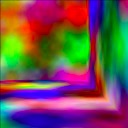##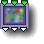33 3D Color MultiFractal

parameters :
A : Amplitude (0. : 2.)
B : Phase (-32. : 32.)
C : Frequency (0. : 16.)
D : Roughness (0.25 : 0.95)

discussion :
3D RGB fractal noise with varying fractal dimension (roughness) created by summing 3D Perlin noise functions at increasing frequencies for each RGB channel. It can be seen as a 3D vector version of the 3D Multifractal noise with an offset to avoid negative colors. It can be used as a multifractal displacement function when it is connected at the top of a three input system.##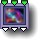33 3D Caustics

parameters :
A : Amplitude (0. : 4.)
B : Phase (-32. : 32.)
C : Frequency (0. : 16.)

discussion :
A 3D fractal vector function that simulates caustics. Caustics are the patterns formed when a point light source is reflected or refracted by a curved surface -- such as one sees on the bottom of a swimming pool on a sunny day. This component is based on 3 independent 3D Ridged Perlin noise. It generates RGB caustic-like shapes. See
21 Ridged noise # You can use the Colorize component to modify the colors to give a bluish-watery caustic look. The Phase parameter can be used to create convincing, natural-looking animation.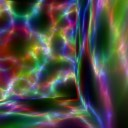##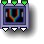33 Packed RGB Crossfade

parameters :
A : Interpolate AB (0. : 1.)
B : Interpolate C (0. : 1.)

discussion :
Crossfade between three RGB images. See the description of the 23 version for a more detailed description. The parameters determine the contribution that the inputs make to the final output.

##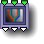33 Packed RGB Alpha

parameters :
A : A Threshold (-1. : 1.)
B : B Feather (0. : 1.)

discussion :
Mix two RGB-based images while applying an alpha (transparency) mask provided by the third input. The third input will usually be a simple value (non-packed stream) and acts as an alpha channel for masking the inputs. If the third input is a packed stream then the effect is a complex mixing of the first two images treating the R, G and B layers of the third image as masks to apply to the red, green and blue layers separately. If input x or y is not packed it will be treated as a gray shade.

Options specifies how infinity is handled:

• "Infinity Intersect (legacy)" - Infinity is transmitted when all inputs are infinity.
• "Keep Infinity in Z" - Infinity is transmitted when input Z is infinity (or input A & B). This is useful to keep the infinity implicit masking whenever z is infinite.

##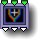33 Packed RGB Add

parameters :
A : Amplitude A (0. : 2.)
B : Amplitude B (0. : 2.)
C : Amplitude C (0. : 2.)

discussion :
Perform an additive weighted mix of the 3 inputs. If any input is not packed it will be treated as a gray shade. It is similar with the
21 S:P Maths Add function on two inputs, except it outputs the RGB summed values separately and works on 3 inputs. "Amplitude A,B,C" scales the inputs prior summation. Infinity in any of the input will make the result infinity.

##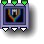33 Packed RGB Max

parameters :
A : Amplitude A (0. : 2.)
B : Amplitude B (0. : 2.)
C : Amplitude C (0. : 2.)

discussion :
Compares the RGB values of all inputs in parallel and returns the highest. "Amplitude A,B,C" scales the inputs prior comparison.
Packed RGB Max can be used as 6 logical MAX performed in parallel if the input vectors are not RGB.

##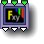33 Packed Maths #

parameters :
A : Amplitude x (0. : 1.)
B : Amplitude y (0. : 1.)
C : Amplitude z (-1. : 1.)

algorithms :
Weighted addition of X, Y and Z. Unlike Packed RGB Add, parameter C 'Amplitude z' can go negative so you may use it to substract Z.
• Blend: ((X:Y):Z)
Crossfade X and Y then fade Z to the result. Parameter A and B controls the fades. This mode is equivalent to Packed RGB Crossfade
• Multiply: (X*Y*Z)
Multiplies all channels. Parameter C 'blend' controls the weight of the multiplication while 'Add' offsets the level of Y & Z prior multiplication. When 'blend' is zero output is X only. When B & C are 1 and Parameter A is 0 output will be Y*Z.
• Add Y & Mul Z: ((X+Y)*Z)
Perform an additive mix of inputs X and Y and filter the result with input Z. Parameters A and B controls the mix of inputs X and Y. Parameter C determines how much filtering Z provides.
• Mul Y & Add Z: ((X*Y)+Z)
As a mixer, this function is often used for global illumination implementation where Y is the light that multiplies the X texture and Z the added specular highlights and reflections. Parameter B controls how much Y multiplies X while Parameter C controls how much Z is added to the mix.
• Blend XY by Z: (X+ Z*(Y-X))
This new operator blends all RGB channels of X & Y with Z(xyz) values in parallel. It can be seen as 3 independent linear interpolation function with Z providing the interpolator values.
Parameter 'Blend z' allows to further blend the interpolator into the mix. if Z is scalar "Blend XY by Z" is equivalent to the 33 Packed RGB Alpha component.
Note that Z<=0 result in X only while Z>=1 result in Y only.

##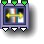33 Packed random Mix

parameters :
A : Amount (0. : 2.)
B : Phase (-32. : 32.)
C : Frequency (0. : 32.)

discussion :
Packed Random Mix takes three RGB packed streams and randomly mixes them using a low frequency built-in random noise function. Use the 34 equivalent
34 Packed random Mix when the alpha/elevation is required like when designing terrains for landscapes rendering in ArtMatic Voyager
"Phase" and 'Frequency' adjusts the mixing noise while 'Amount' controls how much input B & C will be mixed in.

##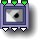33 Packed XYZ Depth sort

parameters :
A : z offset (-16. : 16.)

discussion :
This component mixes three 3D objects into a single set of 3D outputs. The inputs should be the packed outputs of 3D objects. The algorithm is such that for each set of points received, the set with the lowest value of z wins; in other words, the coordinates closest to the viewer win.

##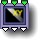33 Packed XYA logic #

parameters :
A : Smoothness % (0. : 16.)

discussion :
Useful in 2D geometry applications, this components return a point (x,y,z) based on the z comparison of 3 packed 3D vectors A,B,C. If a scalar is sent to input 3 (Cz) its value will affect the z output only that will be the max of (Az,Bz,Cz).
The returned point may be either of the input depending on the chosen algorithm. 'Smoothness' parameter will blend the transitions between 2D spaces if non zero. 33 Packed XYA logic can be used to create 2D IFS fractals and 2D graphics by splitting the space according to z values.

algorithms :
• Maximum z(alpha):
return the point with maximum z
• Minimum z(alpha):
return the point with minimum z
• Overlay :
Use the "Overlay" logic applied to points
• Underlay :
Use the "Underlay" logic applied to points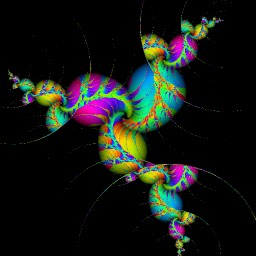IFS fractal based on XYA logic. Example file : Libraries/Components demo/XYA logic IFS

##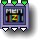33 Memory Packed Alpha

parameters :
A : A Threshold (-1. : 1.)
B : B Feather (0. : 1.)

discussion :
This is an accumulating version of the
Packed RGB Alpha component designed to be used in iterated trees. Usually, inputs 1 and 2 will be packed RGB streams and input 3 will be a simple value acting as an alpha channel mask. Adjusting the 'Threshold' and 'Feather' can have a dramatic effect when using this component as it adjust the alpha level and contrast. Keep the feather at 1 and level at zero to leave the incoming alpha unchanged.

##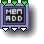33 Memory Add

parameters :
A : Start Value (-4. : 2.)
B : Sum Gain (0. : 2.)
C : Auto Gain (0. : 1.)

discussion :
This component adds the vector (x,y,z) of each iteration to the accumulated values initialized with the parameter "Start value". It is basically a summation over iterations and the output sum vector is scaled by 'Sum Gain' parameter.
When 'Auto Gain' is set at 1 the weight will be calculated using the context iteration max to keep the sum independent of the iteration number. Lower values will blend with the 'Sum Gain' setting.
Incoming infinities are discarded and treated as transparent.

##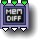33 Memory Diff

parameters :
No parameters.

discussion :
Memory Diff performs the absolute value of the difference between input vector and stored values initialized at (0,0,0).

##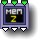33 Memory Depth sort

parameters :
No parameters.

discussion :
This component is a memory-based version of the other depth sort functions. Rather than performing a depth sort between two sets of inputs, the depth sort is performed between the current set of values and the stored values from the previous iteration. Depth Sort preserves the values that correspond to the frontmost pixel when the three values are considered as 3D position. The frontmost pixel corresponds to the value with the lowest valued z (third) coordinate. This component is useful for accumulating pseudo 3D objects in recursive and iterative trees.
Example: Libraries/Components demo/Ammonite

## 33 Compiled Tree :

parameters :
A : Blend 0:1
B : Recursions

discussion :
Compiled trees are groups of tiles that can be used in place of single tiles as a kind of macro or subroutine. 33 CTs can hold groups of 3D space transformations or can be used to manipulate 3 streams of datas : scalar single values or 3D or 4D packed vectors.

usage :
Select a 33 tile and use "New compiled tree" to create a new CT from the selection (Tree Edit menu or type 'n' key).
To save a CT on disk to use the function elsewhere use "Save compiled tree" from the Tree Edit menu.
You may also copy and paste the entire CT by using Copy Tile and Paste Tile from the Edit menu.

CTs can be used recursively if the option "Allow feedback" is set. In that case output is fed to the input at second iteration and the transform will be applied "N" times, N being set by the "Recursions" parameter.
When recursed the type of input shall match the type of ouputs. It is possible to recurse 2D coordinates in XY while using a packed RGBA stream for input3. The CT has then to accumulate the color+alpha data that will be sent to third output using a Memory tile (see
44 Memory w Logic # discussion).
33 CTs can also (ArtMatic Engine 8.0) recurse a packed RGBA stream in third input/output. In that case you may also use S:P Logic &Profiles to accumulate the RGBA data within the CT. In both ways you can create advanced color-shaded 2D fractals using 33 Compiled Tree.